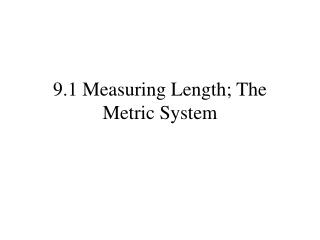DownloadDownload Presentation9.1 Measuring Length; The Metric System

# 9.1 Measuring Length; The Metric System

Télécharger la présentation## 9.1 Measuring Length; The Metric System

- - - - - - - - - - - - - - - - - - - - - - - - - - - E N D - - - - - - - - - - - - - - - - - - - - - - - - - - -
##### Presentation Transcript

1. 9.1 Measuring Length; The Metric System

2. Every measurement needs to have a number and a unit of measure. Stating that something has a length of 3 is meaningless. It could be 3 feet, 3 meters, 3 light years, 3 rods… The linear units of measure in the English system are: 12 inches = 1 foot 3 feet = 1 yard 5,280 feet = 1 mile (A roman mile was 1,000 paces or 5,000 feet)

3. Measurements can be changed from one unit to another by multiplying by unit fractions. 12 inches = 1 foot 3 feet = 1 yard 5,280 feet = 1 mile 12 inches 1 foot 3 feet 1 yard 1 foot 12 inches 1 yard 3 feet 5280 feet 1 mile 1 mile 5280 feet

4. To convert 3 miles to inches, multiply by unit fractions. 12 inches 1 foot 3 feet 1 yard 1 foot 12 inches 1 yard 3 feet 5280 feet 1 mile 1 mile 5280 feet The miles and feet will cancel leaving inches as the unit of measure.

5. To convert 3 inches to miles, multiply by unit fractions. 12 inches 1 foot 3 feet 1 yard 1 foot 12 inches 1 yard 3 feet 5280 feet 1 mile 1 mile 5280 feet The inches and feet will cancel leaving miles as the unit of measure.

6. To convert 4400 yards to miles, multiply by unit fractions. 12 inches 1 foot 3 feet 1 yard 1 foot 12 inches 1 yard 3 feet 5280 feet 1 mile 1 mile 5280 feet The inches and feet will cancel leaving miles as the unit of measure.

7. This chart is found on page 504

8. Common units for linear measure in the metric system This chart is found on page 504

9. The little marks at the top of the ruler are 1 millimeter apart. The units marked at the top are centimeters. 10 millimeters make 1 centimeter. There are 2.54 centimeters in one inch. 2.54 cm = 1 inch

10. 2.54 cm = 1 inch

11. To convert units within the metric system, multiply or divide by 10. Multiply by 10 as the units go to the right. Divide by 10 as the units go to the left. 0.1 km =1 hm 0.1 hm =1 dam 0.1 dam =1 m 0.1 m =1 dm 0.1 dm =1 cm 0.1 cm =1 mm km hm dam m dm cm mm 1 km =10 hm 1 hm =10 dam 1 dam =10 m 1 m =10 dm 1 dm =10 cm 1 cm =10 mm

12. Convert 327.8 meters to kilometers Divide by 10 as the units go to the left. km hm dam m dm cm mm

13. Convert 21.7 dekameters to centimeters Multiply by 10 as the units go to the right. km hm dam m dm cm mm

14. Convert 483.2 hectometers to millimeters Multiply by 10 as the units go to the right. km hm dam m dm cm mm

15. Measurements can be changed from one unit to another by multiplying by unit fractions. 1 inches = 2.54 cm 1 foot = 30.48 cm 1 yard ≈ 0.9 meter 1 mile ≈ 1.6 kilometers 1 inch 2.54 cm 1 foot 30.48 cm 2.54 cm 1 inch 30.48 cm 1 foot 1 yard 0.9 meter 0.9 meter 1 yard 1 mile 1.6 km 1.6 km 1 mile

16. 1 inch 2.54 cm 1 foot 30.48 cm 2.54 cm 1 inch 30.48 cm 1 foot 1 yard 0.9 meter 0.9 meter 1 yard 1 mile 1.6 km 1.6 km 1 mile Convert 225 miles to kilometers.

17. 1 inch 2.54 cm 1 foot 30.48 cm 2.54 cm 1 inch 30.48 cm 1 foot 1 yard 0.9 meter 0.9 meter 1 yard 1 mile 1.6 km 1.6 km 1 mile Convert 44 yards to meters.

18. 1 inch 2.54 cm 1 foot 30.48 cm 2.54 cm 1 inch 30.48 cm 1 foot 1 yard 0.9 meter 0.9 meter 1 yard 1 mile 1.6 km 1.6 km 1 mile Convert 44 meters to yards.

19. 1 inch 2.54 cm 1 foot 30.48 cm 2.54 cm 1 inch 30.48 cm 1 foot 1 yard 0.9 meter 0.9 meter 1 yard 1 mile 1.6 km 1.6 km 1 mile Convert 34 inches to centimeters.

20. 1 inch 2.54 cm 1 foot 30.48 cm 2.54 cm 1 inch 30.48 cm 1 foot 1 yard 0.9 meter 0.9 meter 1 yard 1 mile 1.6 km 1.6 km 1 mile Convert 75 miles per hour to kilometers per minute.

21. 1 inch 2.54 cm 1 foot 30.48 cm 2.54 cm 1 inch 30.48 cm 1 foot 1 yard 0.9 meter 0.9 meter 1 yard 1 mile 1.6 km 1.6 km 1 mile Convert 15 centimeters per second to miles per hour.